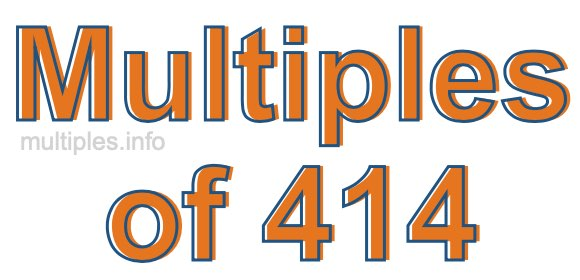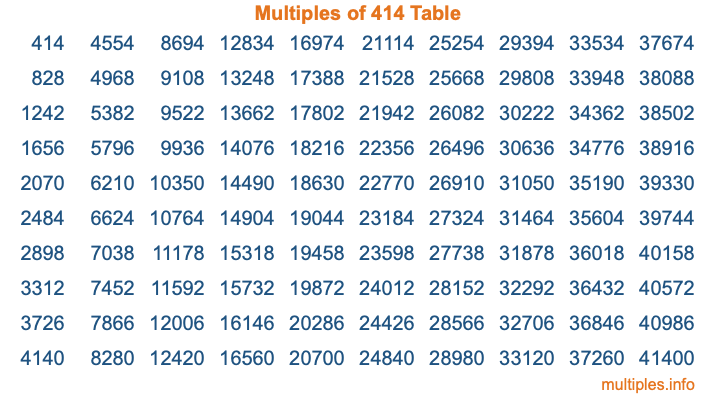Multiples of 414Welcome to the Multiples of 414 page. Here we will first teach you everything you will ever need to know about the multiples of 414, and then give you a study guide summary of everything we taught you to make sure you remember it all. Use this page to look up facts and learn information about the multiples of 414. This page will make you a multiples of four hundred fourteen expert!

Definition of Multiples of 414
Multiples of 414 are all the numbers that when divided by 414 equal an integer. Each of the multiples of 414 are called a multiple. A multiple of 414 is created by multiplying 414 by an integer.

Therefore, to create a list of multiples of 414, you start with 1 multiplied by 414, then 2 multiplied by 414, then 3 multiplied by 414, and so on for as long as you want. Thus, the list of the first five multiples of 414 is 414, 828, 1242, 1656, and 2070. To see a larger list of multiples of 414, see the printable image of Multiples of 414 further down on this page. We also have a category where you can choose any nth multiple of 414.

Multiples of 414 Checker
The Multiples of 414 Checker below checks to see if any number of your choice is a multiple of 414. In other words, it checks to see if there is any number (integer) that when multiplied by 414 will equal your number. To do that, we divide your number by 414. If the the quotient is an integer, then your number is a multiple of 414.

Is  a multiple of 414?

Least Common Multiple of 414 and ...
A Least Common Multiple (LCM) is the lowest multiple that two or more numbers have in common. This is also called the smallest common multiple or lowest common multiple and is useful to know when you are adding our subtracting fractions. Enter one or more numbers below (414 is already entered) to find the LCM.

Check out our LCM Calculator if you need more details about the Least Common Multiple or if you need the LCM for different numbers for adding and subtraction fractions.

nth Multiple of 414
As we stated above, 414 is the first multiple of 414, 828 is the second multiple of 414, 1242 is the third multiple of 414, and so on. Enter a number below to find the nth multiple of 414.

th multiple of 414

Multiples of 414 vs Factors of 414
414 is a multiple of 414 and a factor of 414, but that is where the similarities end. All postive multiples of 414 are 414 or greater than 414. All positive factors of 414 are 414 or less than 414.

Below is the beginning list of multiples of 414 and the factors of 414 so you can compare:

Multiples of 414: 414, 828, 1242, 1656, 2070, etc.

Factors of 414: 1, 2, 3, 6, 9, 18, 23, 46, 69, 138, 207, 414

As you can see, the multiples of 414 are all the numbers that you can divide by 414 to get a whole number. The factors of 414, on the other hand, are all the whole numbers that you can multiply by another whole number to get 414.

It's also interesting to note that if a number (x) is a factor of 414, then 414 will also be a multiple of that number (x).

Multiples of 414 vs Divisors of 414
The divisors of 414 are all the integers that 414 can be divided by evenly. Below is a list of the divisors of 414.

Divisors of 414: 1, 2, 3, 6, 9, 18, 23, 46, 69, 138, 207, 414

The interesting thing to note here is that if you take any multiple of 414 and divide it by a divisor of 414, you will see that the quotient is an integer.

Multiples of 414 Table
Below is an image of the first 100 multiples of 414 in a table. The table is in chronological order, column by column. The first column has the first ten multiples of 414, the second column has the next ten multiples of 414, and so on.The Multiples of 414 Table is also referred to as the 414 Times Table or Times Table of 414. You are welcome to print out our table for your studies.

Negative Multiples of 414
Although not often discussed or needed in math, it is worth mentioning that you can make a list of negative multiples of 414 by multiplying 414 by -1, then by -2, then by -3, and so on, to get the following list of negative multiples of 414:

-414, -828, -1242, -1656, -2070, etc.

Multiples of 414 Summary
Below is a summary of important Multiples of 414 facts that we have discussed on this page. To retain the knowledge on this page, we recommend that you read through the summary and explain to yourself or a study partner why they hold true.

There are an infinite number of multiples of 414.

A multiple of 414 divided by 414 will equal a whole number.

414 divided by a factor of 414 equals a divisor of 414.

The nth multiple of 414 is n times 414.

The largest factor of 414 is equal to the first positive multiple of 414.

414 is a multiple of every factor of 414.

414 is a multiple of 414.

A multiple of 414 divided by a divisor of 414 equals an integer.

414 divided by a divisor of 414 equals a factor of 414.

Any integer times 414 will equal a multiple of 414.

Multiples of a Number
Here you can get the multiples of another number, all with the same attention to detail as we did for multiples of 414 on this page.

Multiples of
Multiples of 415
Did you find our page about multiples of four hundred fourteen educational? Do you want more knowledge? Check out the multiples of the next number on our list!